# I.E. Irodov Solutions on Liquids - Capillary Effects

Liquids flow because the intermolecular forces between molecules are weak enough to allow the molecules to move around relative to one another. Also, we can define capillary action as a phenomenon where ascension of liquids through a tube or cylinder takes place. This primarily occurs due to adhesive and cohesive forces. Students can access solutions on liquid capillary effects questions here. All questions listed here are solved by subject experts asked in I.E. Irodov book PART TWO. THERMODYNAMICS AND MOLECULAR PHYSICS - 2.5. Liquids. Capillary Effects. Each solution is explained stepwise and with formulas, equations, graphs, and diagrams wherever required. Students aiming for a high score in competitive examination must solve all the given problems for easy understanding and to have a grip over the syllabus and concept.

### I.E. Irodov Solutions on Liquids - Capillary Effects

1. Find the capillary pressure

1. (a) in mercury droplets of diameter d = 1.5 pm;

2. (b) inside a soap bubble of diameter d = 3.0 mm if the surface tension of the soap water solution is α = 45 mN/m.

Solution:

1. (a) $\Delta p = \alpha(\frac{1}{d/2}+\frac{1}{d/2}) = \frac{4 \alpha}{d}$

= [4x490x10-3]/[1.5x10-6] N/m2

= 1.307 x 106 N/m2 = 13 atm

(b) The soap bubble has two surfaces

$\Delta p = 2 \alpha(\frac{1}{d/2}+\frac{1}{d/2}) = \frac{8 \alpha}{d}$

= [8x45x10-3]/[3x10-3]

= 1.2 x 10-3 atm

2. In the bottom of a vessel with mercury there is a round hole of diameter d = 70 μm. At what maximum thickness of the mercury layer will the liquid still not flow out through this hole?

Solution:

1. The pressure just inside the hole will be less than the outside pressure by 4 α/d. This can support a height h of Hg.

ρgh = 4α/d or h = 4α/ρgd

= [4x490x10-3]/[13.6x103x9.8x70x10-6]

= 0.21 m of Hg (approx.)

3. A vessel filled with air under pressure P0 contains a soap bubble of diameter d. The air pressure having been reduced isothermally n-fold, the bubble diameter increased η-fold. Find the surface tension of the soap water solution.

Solution:

1. By Boyle's law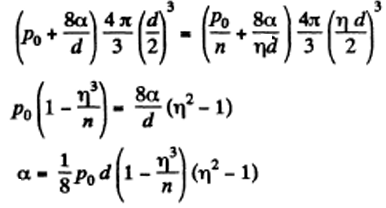4. Find the pressure in an air bubble of diameter d = 4.0 μm, located in water at a depth h = 5.0 m. The atmospheric pressure has the standard value P0.

Solution:

1. The pressure has terms due to hydrostatic pressure and capillarity and they add

p = p0 + ρgh + 4α/d

= (1 + [5x9.8x103]/105 + [4x0.73x10-3]/[4x10-6] x 10-5) atoms

= 2.22 atom

5. The diameter of a gas bubble formed at the bottom of a pond is d = 4.0 μm. When the bubble rises to the surface its diameter increases n = 1.1 times. Find how deep is the pond at that spot. The atmospheric pressure is standard, the gas expansion is assumed to be isothermal.

Solution:

1. By Boyle's law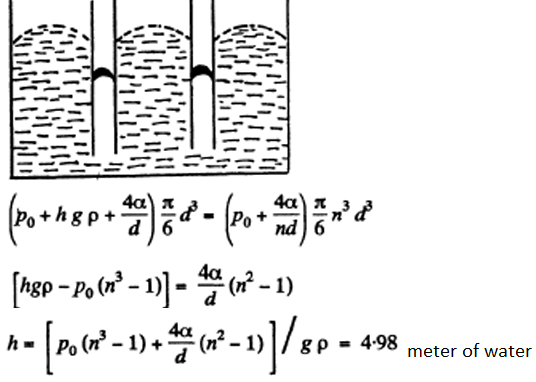6. Find the difference in height of mercury columns in two communicating vertical capillaries whose diameters are d1 = 0.50 mm and d2 = 1.00 mm, if the contact angle θ = 138o.

Solution:

1. $\Delta h \rho g = 4 \alpha |cos \theta|(\frac{1}{d_1}-\frac{1}{d_2})$

or ∆h = 11 mm

7. A vertical capillary with inside diameter 0.50 mm is submerged into water so that the length of its part protruding over the water surface is equal to h = 25 mm. Find the curvature radius of the meniscus.

Solution:

1. In a capillary with diameter d = 0.5 mm water will rise to a height.

2α/ρgr = 4α/ρgd = 59.6 mm

Since this is greater than the height (= 25 mm) of the tube, a meniscus of radius R will be formed at the top of the tube, where R = 2α/ρgh = 0.6 mm (approx.).

8. A glass capillary of length l = 110 mm and inside diameter d = 20 μm is submerged vertically into water. The upper end of the capillary is sealed. The outside pressure is standard. To what length x has the capillary to be submerged to make the water levels inside and outside the capillary coincide?

Solution:

1. Initially the pressure of air in the capillary is p0 and it’s length is Z. When submerged under water, the pressure of air in the portion above water must be (po + 4α/d), since the level of water inside the capillary is the same as the level outside.

Thus, by Boyle’s law

(po + 4α/d)(l - x) = pol

(4α/d)(l - x) = pox

Or x = l/(1 + (pod/4 α))

9. When a vertical capillary of length l with the sealed upper end was brought in contact with the surface of a liquid, the level of this liquid rose to the height h. The liquid density is p, the inside diameter of the capillary is d, the contact angle is θ, the atmospheric pressure is P0. Find the surface tension of the liquid.

Solution:

1. By Boyle’s law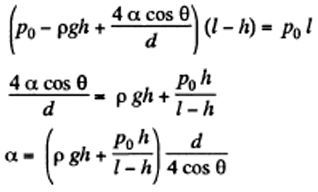10. A glass rod of diameter d1 = 1.5 mm is inserted symmetrically into a glass capillary with inside diameter d2 = 2.0 mm. Then the whole arrangement is vertically oriented and brought in contact with the surface of water. To what height will the water rise in the capillary?

Solution:

1. Suppose the liquid rises to a height h. Then the total energy of the liquid in the capillary is

$E(h) = \frac{\pi}{4}(d_1^2- d_2^2)h \times \rho g \times \frac{h}{2}- \pi(d_2-d_1)\alpha h$

Minimising E, we get

h = 4α/[ ρg(d2-d1)] = 6 cm.

11. Two vertical plates submerged partially in a wetting liquid form a wedge with a very small angle δφ. The edge of this wedge is vertical. The density of the liquid is p, its surface tension is α, the contact angle is θ. Find the height h, to which the liquid rises, as a function of the distance x from the edge.

Solution:

1. Let h be the height of the water level at a distance x from the edge. Then the total energy of water in the wedge above the level outside is,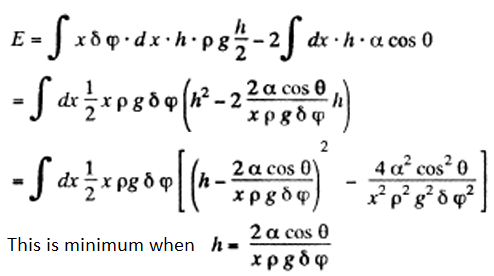12. A vertical water jet flows out of a round hole. One of the horizontal sections of the jet has the diameter d = 2.0 mm while the other section located l = 20 mm lower has the diameter which is n = 1.5 times less. Find the volume of the water flowing from the hole each second.

Solution:

1. From the equation of continuity

$\frac{\pi}{4}d^2v = \frac{\pi}{4}(\frac{d}{n})^2V$

or V = n2v

We then apply Bernoulli’s theorem

p/ρ + (1/2)v2 + ϕ = constant

The pressure p differs from the atmospheric pressure by capillary effects. At the upper section :

p =po + 2α/d

Neglecting the curvature in the vertical plane. Thus,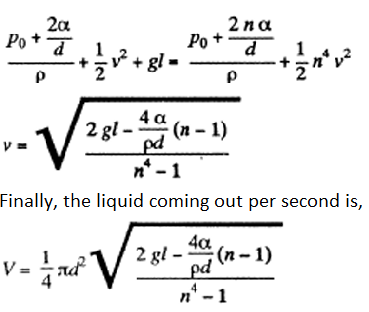13. A water drop falls in air with a uniform velocity. Find the difference between the curvature radii of the drop's surface at the upper and lower points of the drop separated by the distance h = 2.3 mm.

Solution:

1. The radius of curvature of the drop is R1 at the upper end of the drop and R2 at the lower end. Then the pressure inside the drop is po + 2α/R1 at the top end and po + 2α/R2 at the bottom end.

Hence, po + 2α/R1 = po + 2α/R2 + ρgh

Or [2α(R2 - R1)]/(R2 R1) = ρgh

To a first approximation, R1 = R2 = h/2

R2 - R1 = (1/8) ρgh3/ α = 0.20 mm(approx..)

If h = 2.3 mm, α = 73 mN/m

14. A mercury drop shaped as a round tablet of radius R and thickness h is located between two horizontal glass plates. Assuming that h <<R, find the mass m of a weight which has to be placed on the upper plate to diminish the distance between the plates n-times. The contact angle equals θ. Calculate m if R = 2.0 cm, h = 0.38 mm, n = 2.0, and θ = 135°.

Solution:

1. Let us first calculate the pressure difference inside the film from that outside.

p = α(1/r1 +1/r2)

Here 2r1|cos θ| = h and r2 = -R (approx) the radius of the tablet and can be neglected. Thus, the total force exerted by mercury drop on the upper glass plate is

[2𝜋 α R2|cos θ|/h

Put h/n for h because the tablet is compressed n times. Then since Hg is nearly, in compressible, 𝜋R2h = constant so R ->R√n.

Total force = [2𝜋 α R2|cos θ|n2/h

Part of the force is needed to keep the Hg in the shape of a table rather than in the shape of infinitely thin sheet. This part can be calculated being putting n = 1 above.

Thus, mg + [2𝜋 α R2|cos θ|/h = [2𝜋 α R2|cos θ|n2/h

Or m = [2𝜋 α R2|cos θ|(n2-1)/hg = 0.7 kg

15. Find the attraction force between two parallel glass plates, separated by a distance h = 0.10 mm, after a water drop of mass m = 70 mg was introduced between them. The wetting is assumed to be complete.

Solution:

1. The pressure inside the film is less than that outside by an amount α(1/r1 + 1/r2) where r1 and r2 are the principal radii of curvature of the meniscus. One of these is small being given by h = 2r1 cosθ while the other is large and will be ignored.

Then , F = α (2A cosθ)/h

where A = area of the water film between the plates.

Now, A = m/ρh

so, F = 2mα/ρh2 when θ = 0.

16. Two glass discs of radius R = 5.0 cm were wetted with water and put together so that the thickness of the water layer between them was h = 1.9 p.m. Assuming the wetting to he completes, find the force that has to be applied at right angles to the plates in order to pull them apart.

Solution:

1. This is analogous to previous problem, except A = πR2

so, F = 2πR2α/h = 0.6 kN

17. Two vertical parallel glass plates are partially submerged in water. The distance between the plates is d = 0.10 mm, and their width is l = 12 cm. Assuming that the water between the plates does not reach the upper edges of the plates and that the wetting is complete, find the force of their mutual attraction.

Solution:

1. The energy of the liquid between the plates is

E = ldhρg (h/2) - 2αlh = (1/2)ρgldh2 - 2αlh

=$\frac{1}{2}\rho gld(h- \frac{2 \alpha}{\rho gd})^2 -\frac{2 \alpha^2 l}{\rho gd}$

This energy is minimum when, h = 2α/ρgd and the minimum potential energy is the Emin = -(2α2l)/ρgd

The force of attraction between the plates can be obtained from this as ,

F = $-\frac{\partial E_{min}}{\partial d}=-\frac{2 \alpha^2l}{\rho g d^2}$

(Minus sign means the force is attractive)

Therefore, F = - αlh/d = 13N

18. Find the lifetime of a soap bubble of radius R connected with the atmosphere through a capillary of length l and inside radius r. The surface tension is α, the viscosity coefficient of the gas is η.

Solution:

1. Suppose the radius of the bubble is x at some instant. Then the pressure inside is p0 + 4α/x.

The flow through the capillary is by Poiseuille’s equation,

$Q = \frac{\pi r^4}{8 \eta l} \frac{4 \alpha}{x} = -4\pi^2 \frac{dx}{dt}$

Integrating $\frac{\pi r^4 \alpha}{2 \eta l} t = \pi(R^4-x^4)$

where we have used the fact that t = 0 where x = R.

This gives, t = [2ηIR4]/[ αr4] as the life time of the bubble corresponding to x = 0.

19. A vertical capillary is brought in contact with the water surface. What amount of heat is liberated while the water rises along the capillary? The wetting is assumed to be complete, the surface tension equals α.

Solution:

1. If the liquid rises to a height h', the energy of the liquid column becomes

E = 𝜋r2ρgh .(h/2) - 2𝜋rhα = (1/2)g 𝜋ρ(rh – 2 x (α/ρg)2 - 2𝜋 α2/ρg

This is minimum when rh =2 α/ρg and that is relevant height to which water must rise.

At this point, Emin = -2𝜋 α2/ρg

Since E = 0 in the absence of surface tension a heat Q = 2𝜋 α2/ρg must have been liberated.

20. Find the free energy of the surface layer of

1. (a) a mercury droplet of diameter d = 1.4 mm;

2. (b) a soap bubble of diameter d = 6.0 mm if the surface tension of the soap water solution is equal to α = 45 mN/m.

Solution:

1. (a) The free energy per unit area being α, F = 𝜋αd2 = 3μJ

(b) F = 2𝜋αd2, because the soap bubble has two surfaces.

Substitution gives F = 10μJ.